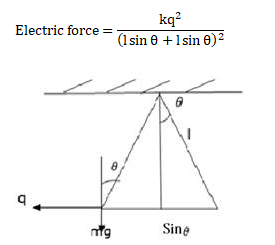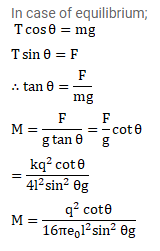# Two identical pith balls, each carrying a charge q,`
Question:

Two identical pith balls, each carrying a charge q, are suspended from a common point by two strings of equal length I. Find the mass of each ball if the angle between the strings is $2 \theta$ in equilibrium.

Solution: